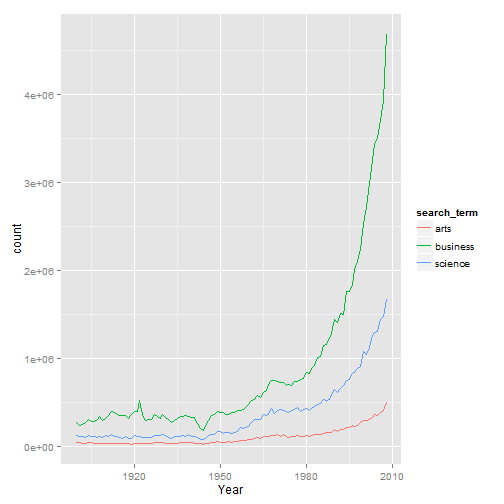Google scans books as a part of its Google Books service. The aim of the service is to allow people to search the content of books, ultimately to facilitate book sales.

As a byproduct of its scanning efforts is the generation of a large corpus of words that it makes available to the public. You can get an estimate of how often a word was used in print during a particular year. A wikipedia article explaining the service is here:

Google provides a website to graph word usage over time:

If you wanted to look only at select words, there is an R package to download the data. We'll cover how to do that in this tutorial.

``````library(ngramr)
``````

## Create a dataframe to house the data.

We will first create a dataframe to house the data. We will start off with a dataframe that has one column, and 109 rows - equal to the number of years from 1900 to 2008.

``````data <- as.data.frame(matrix(ncol=1, nrow=109))
``````

## Create a column of years.

Next, we will list out the years in sequence, and rename the column to “year”

``````data\$V1 <- seq(from=1900, to=2008)

names(data)[names(data)=="V1"] <- "Year"
``````

## Specify the terms to search for in Google Ngram.

``````search_terms <- c("arts", "business", "science")
``````

## Loop through those search terms and pull in Jstor data.

``````# Create a loop
for(i in 1:length(search_terms)){

# Get each search term and store those in objects
term <- search_terms[i]

# Search for the term in the English 2012 corpus, starting from the year 1900 to 2008
# Then house the output in a dataframe
temp <- ngram(term, corpus = "eng_2012", year_start = 1900, smoothing = 0,
count = T, tag = NULL, case_ins = FALSE)

# Merge NYT data with dataframe created step 1, matching by years
data <- merge(data, temp[,c("Year", "Count")], by ="Year", all.x=TRUE)

# Reaname column by search term
colname <- paste(term, sep="")

# Rename added column with ID
names(data)[names(data)=="Count"] <- colname

# Remove temporary dataframe
rm(temp)

}

# Rename the year variable from "term" to "year"
#names(data)[names(data)=="term"] <- "year"
``````

A look at the data.

``````head(data)
``````
``````##   Year  arts business science
## 1 1900 36155   265231  119665
## 2 1901 38291   235711  104036
## 3 1902 33719   250901  106028
## 4 1903 28753   266980   97765
## 5 1904 36403   298290  121023
## 6 1905 35930   275559  110596
``````

## Reshape the data to put it in a graph friendly (long) format.

``````data_long <- reshape(data,
v.names = "count",
timevar = "search_term",
direction = "long")
``````

## Graph the data to observe trends over time.

As you can see from the graph below, the term “business” has been mentioned to a greater extent than “arts” or “science”, but the disparity in use has accelerated with time. One thing to carefully think through when analysing word count data are possible collisions in meaning. The term “business” could refer a for-profit organization, or one's state of affairs, as in “mind your own business”. With only word counts, there is no way to disentangle the two uses of the meaning.``````library(ggplot2)

p <- ggplot(data_long, aes(x=Year, y=count, group=search_term))

p +  geom_line(aes(colour = search_term))
``````